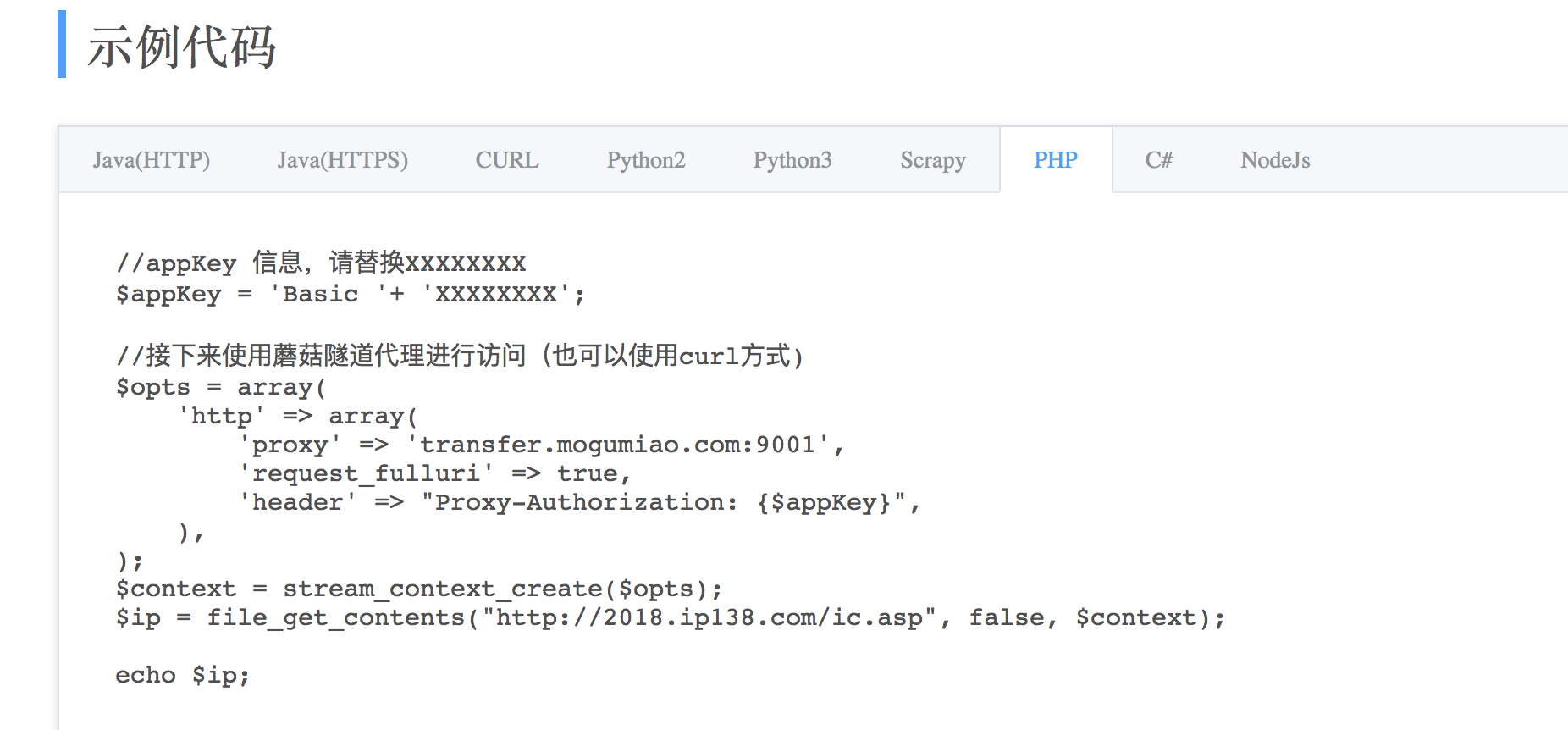使用代理ip防止爬虫被封ip(附蘑菇代理开发过程)

1、第一种方式

$ch = curl_init(); curl_setopt($ch, CURLOPT_URL, $link);//要访问的url curl_setopt ($ch, CURLOPT_PROXY, $ip);//使用代理访问$ip是 ip:port 格式
curl_setopt($ch,CURLOPT_USERAGENT,'Mozilla/5.0 (Windows; U; Windows NT 5.1; en-US; rv:1.8.1.13) Gecko/20080311 Firefox/3.0.0.13');//有的网站需要ua，设置一下 curl_setopt($ch,CURLOPT_HEADER,0);
curl_setopt($ch,CURLOPT_RETURNTRANSFER,1); curl_setopt($ch, CURLOPT_SSL_VERIFYPEER, false);//https网站取消ssl验证
curl_setopt($ch,CURLOPT_FOLLOWLOCATION,1);//允许30*跳转 curl_setopt($ch, CURLOPT_TIMEOUT,30);//设置超时时间
$response = curl_exec($ch);
if ($response === false) {$error_info =  curl_error($ch); curl_close($ch);//关闭curl
return false;
} else {
curl_close($ch);//关闭curl var_dump($response);
}

2、第二种方式$appKey = 'Basic '. 'Q2R1cmNFbEc1VWU5eGVKYTpqcUZmNU';//这里appkey在蘑菇代理控制台找到$ch = curl_init();
curl_setopt($ch, CURLOPT_URL,$link);//要访问的url
curl_setopt ($ch, CURLOPT_PROXY, 'transfer.mogumiao.com:9001');//使用代理访问 curl_setopt($ch,CURLOPT_USERAGENT,'Mozilla/5.0 (Windows; U; Windows NT 5.1; en-US; rv:1.8.1.13) Gecko/20080311 Firefox/2.1.0.13');
curl_setopt ($ch, CURLOPT_HTTPHEADER, ["Proxy-Authorization: {$appKey}"]);//设置代理权限
curl_setopt($ch,CURLOPT_RETURNTRANSFER,1); curl_setopt($ch, CURLOPT_SSL_VERIFYPEER, false);//https网站取消ssl验证
curl_setopt($ch,CURLOPT_FOLLOWLOCATION,1);//允许30*跳转 curl_setopt($ch, CURLOPT_TIMEOUT,30);//设置超时时间
$response = curl_exec($ch);
if ($response === false) {$error_info =  curl_error($ch); curl_close($ch);//关闭curl
return false;
} else {
curl_close($ch);//关闭curl var_dump($response);
}